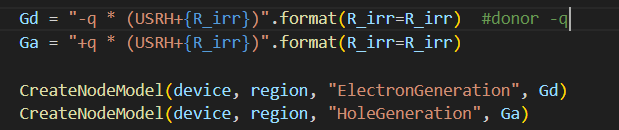# Solver cannot converge at higher rates

Hi all! I’m new here. When I was doing the 1d Silicon diode simulation with higher recombination rates(for example, the rates caused by irradiation defects, which is usually considered as adding an extra recombination rates to the USRH), the solver cannot converge. I checked the output and find out that after some iterations the absolute error will cycle among three values and never converge to 0. I tried to print the node model values of ElectronGeneration and find it reached 2.262e-36. By the way, the ElectronGeneration of usual Si diode simulation (I mean, without adding the defect) is 9.647e-41. Do you have any idea on how to solve this problem?

Hi @Zhan, welcome to the forum!

Would you mind sharing a screenshot of the convergence history of the iterations?

Did you implement the derivatives of your models with respect to the solution variables, USRH:Electrons, USRH:Holes ?

I would not expect the AbsoluteError to ever to be exactly zero. The Relative Error will tell you what the relative change is per iteration. You should see the relative errors go down by orders of magnitude on each iteration if all of the derivatives are implemented properly. For double precision arithmetic, the relative error minimum is usually around 1e-15.

Hi Juan,
Thanks for your reply. Of course I can show the screenshot and log files.

And I put the log file indevsim_output.log. Please tell me if you can access that.
I think I did the implements like this:Thank you.

Hi @Zhan

Please start with `R_irr=0` and see if the simulation converges. Since each simulation depends on the previous solution, then step `R_irr` over a few iterations. You can experiment with varying the value linearly or by orders of magnitude.

The warning on the iterations is that the global parameter for `top_bias` will be ignored and the device parameter will be used instead.

Hi Juan,
Thanks for your reply. I tried several R_irr, and find that at R_irr=1e15 it still can converge successfully. Then I tried R_irr=1e20 it fails to converge. (There is no step here, I directly give the value to R_irr)
I am sorry I am not sure what you mean by step the R_irr. Do you mean that I should use R_irr=0 as a trial solution to get, for example, the R_irr=1e1 result, then use 1e1 as the trial solution to get 1e2 result?
And what would happen if the top_bias was ignored and the device parameter will be used?
Thank you.

Device parameters override global parameters. The global value is ignored so you need to be sure to be varying the device value.

What I recommend is something is changing your model equations to somthing like this:

``````set_parameter(name="R_irr", value=0)
Gd = "-q * (USRH + R_irr)"
Ga = "+q * (USRH + R_irr)"
``````

and then ramp using something like this:

``````for i in (1e15, 1e16, 1e17, 1e18, 1e19, 1e20):
set_parameter(name="R_irr", value=0)
solve(......)
``````

where you need to provide the approriate arguments to the `solve` command.

The solution for each parameter value is used as an initial guess to the later simulations.